Next: Dark and Bright Symmetry Up: Real-Time Symmetry Transform Previous: Intersection of Lines of

## Real-Time Implementation

A particular strength of the interest algorithm proposed above is its efficiency when implemented computationally. The relevant details and limitations of the real-time implementations will be discussed below. For a thorough analysis of the implementation, refer to Sela .

An important limitation to Sela's real-time implementation is its use of binary edge magnitudes. Thus, the gradient map must be thresholded. This process reduces the sensitivity of the computations since the values of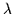(contrast) are limited to 1 bit of dynamic range.

In Equation, the symmetry lines are computed by considering the set of edges in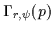. The latter can be visualized as a set of annular regions or rings centered around point p (see Figure). Only pairs of edges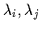from the edge map falling within the ring will be used to compute the symmetry lines at that point. Furthermore, edge magnitudes must be attenuated if their normals are misaligned with the normals of the annular sampling region. The normals of the annular sampling region are merely the normals of its contour as shown in Figure. The contribution of a cocircular edge is proportional to its magnitude projected onto the normals of the annular sampling region . However, Sela deals exclusively with binary values for edge magnitude. Thus, he totally discards the contribution of edges whose phase angle is not within a range of the normal of the annular sampling region they fall into. His computation only considers edges whose phase is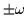degrees from the normal of the annular sampling region instead of gradually attenuating the contribution of misaligned edges. A value of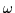=6 degrees is typical. Thus, only edges somewhat parallel or anti-parallel with the normal of the annular sampling region they fall into are used in computing cocircularity. Figuredepicts the filtering performed to discard (instead of attenuate) the misaligned edges.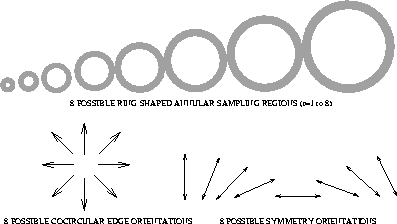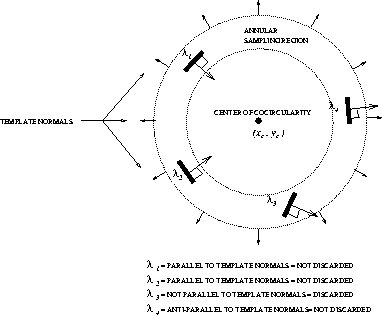In Sela , the dynamic range of r is limited to 3 bits as is the dynamic range of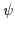. In other words, only 8 different symmetry orientations at 8 different scales (sampling rings) are computed (see Figure). Furthermore, the phase description of the cocircular edges is also limited to one of 8 possible values. The reduction of dynamic range enables Sela to utilize pre-computed lookup tables to obtain the symmetry and interest maps instead of performing detailed calculations during the execution of the transform.Next: Dark and Bright Symmetry Up: Real-Time Symmetry Transform Previous: Intersection of Lines of
Tony Jebara
2000-06-23